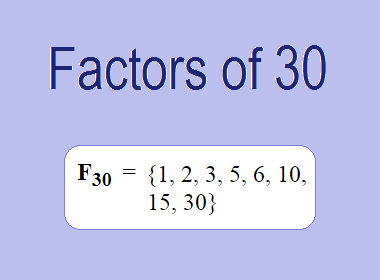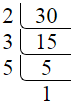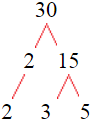# Factors of 30The factors of 30 are 1, 2, 3, 5, 6, 10, 15, and 30 i.e. F30 = {1, 2, 3, 5, 6, 10, 15, 30}. The factors of 30 are all the numbers that can divide 30 without leaving a remainder.

We can check if these numbers are factors of 30 by dividing 30 by each of them. If the result is a whole number, then the number is a factor of 30. Let's do this for each of the numbers listed above:

·        1 is a factor of 30 because 30 divided by 1 is 30.

·        2 is a factor of 30 because 30 divided by 2 is 15.

·        3 is a factor of 30 because 30 divided by 3 is 10.

·        5 is a factor of 30 because 30 divided by 5 is 6.

·        6 is a factor of 30 because 30 divided by 6 is 5.

·        10 is a factor of 30 because 30 divided by 10 is 3.

·        15 is a factor of 30 because 30 divided by 15 is 2.

·        30 is a factor of 30 because 30 divided by 30 is 1.

## How to Find Factors of 30?

1 and the number itself are the factors of every number. So, 1 and 30 are two factors of 30. To find the other factors of 30, we can start by dividing 30 by the numbers between 1 and 30. If we divide 30 by 2, we get a remainder of 0. Therefore, 2 is a factor of 30. If we divide 30 by 3, we get a remainder of 0. Therefore, 3 is also a factor of 30.

Next, we can check if 4 is a factor of 30. If we divide 30 by 4, we get a remainder of 2. Therefore, 4 is not a factor of 30. If we divide 30 by 5, we get a remainder of 0. Therefore, 5 is also a factor of 30. We can continue this process for all the possible factors of 30.

Through this process, we can find that the factors of 30 are 1, 2, 3, 5, 6, 10, 15, and 30. These are the only numbers that can divide 30 without leaving a remainder.

********************

********************

## Properties of the Factors of 30

The factors of 30 have some interesting properties. One of the properties is that the sum of the factors of 30 is equal to 72. We can see this by adding all the factors of 30 together:

1 + 2 + 3 + 5 + 6 + 10 + 15 + 30 = 72

Another property of the factors of 30 is that the prime factors of 30 are 2, 3, and 5.

## Applications of the Factors of 30

The factors of 30 have several applications in mathematics. One of the applications is in finding the highest common factor (HCF) of two or more numbers. The HCF is the largest factor that two or more numbers have in common. For example, to find the HCF of 30 and 42, we need to find the factors of both numbers and identify the largest factor they have in common. The factors of 30 are 1, 2, 3, 5, 6, 10, 15, and 30. The factors of 42 are 1, 2, 3, 6, 7, 14, 21, and 42. The largest factor that they have in common is 6. Therefore, the HCF of 30 and 42 is 6.

Another application of the factors of 30 is in prime factorization. Prime factorization is the process of expressing a number as the product of its prime factors. The prime factors of 30 are 2, 3, and 5, since these are the only prime numbers that can divide 30 without leaving a remainder. Therefore, we can express 30 as:

30 = 2 × 3 × 5

We can do prime factorization by division and factor tree method also. Here is the prime factorization of 30 by division method,30 = 2 × 3 × 5

Here is the prime factorization of 30 by the factor tree method,30 = 2 × 3 × 5

## Conclusion

The factors of 30 are the numbers that can divide 30 without leaving a remainder. The factors of 30 are 1, 2, 3, 5, 6, 10, 15, and 30. The factors of 30 have some interesting properties, such as having a sum of 72. The factors of 30 have several applications in mathematics, such as finding the highest common factor and prime factorization.